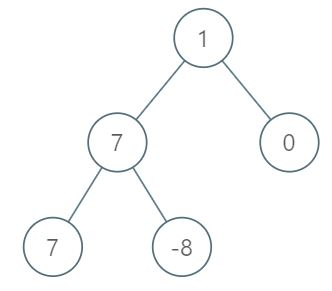## 1161. Maximum Level Sum of a Binary Tree

Given the `root` of a binary tree, the level of its root is `1`, the level of its children is `2`, and so on.

Return the smallest level `x` such that the sum of all the values of nodes at level `x` is maximal.

Example 1:```Input: root = [1,7,0,7,-8,null,null]
Output: 2
Explanation:
Level 1 sum = 1.
Level 2 sum = 7 + 0 = 7.
Level 3 sum = 7 + -8 = -1.
So we return the level with the maximum sum which is level 2.
```

Example 2:

```Input: root = [989,null,10250,98693,-89388,null,null,null,-32127]
Output: 2
```

Constraints:

• The number of nodes in the tree is in the range `[1, 104]`.
• `-105 <= Node.val <= 105`

## Rust Solution

``````struct Solution;
use rustgym_util::*;
use std::collections::HashMap;

trait Preorder {
fn preorder(&self, level: usize, sum: &mut HashMap<usize, i32>);
}

fn preorder(&self, level: usize, sum: &mut HashMap<usize, i32>) {
if let Some(node) = self {
let val = node.borrow().val;
*sum.entry(level).or_default() += val;
let left = &node.borrow().left;
let right = &node.borrow().right;
left.preorder(level + 1, sum);
right.preorder(level + 1, sum);
}
}
}

impl Solution {
fn max_level_sum(root: TreeLink) -> i32 {
let mut sum = HashMap::new();
root.preorder(1, &mut sum);
*sum.iter().max_by_key(|(_, &v)| v).unwrap().0 as i32
}
}

#[test]
fn test() {
let root = tree!(1, tree!(7, tree!(7), tree!(-8)), tree!(0));
let res = 2;
assert_eq!(Solution::max_level_sum(root), res);
}
``````

Having problems with this solution? Click here to submit an issue on github.